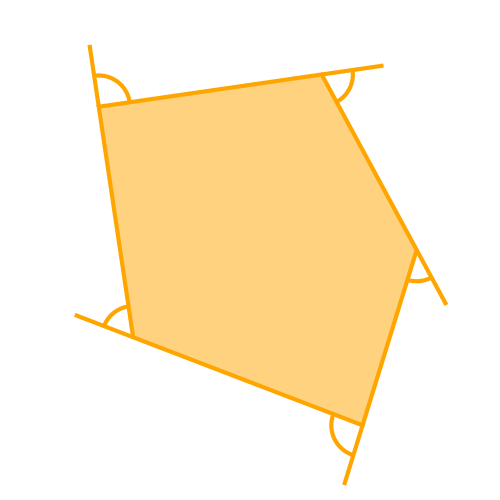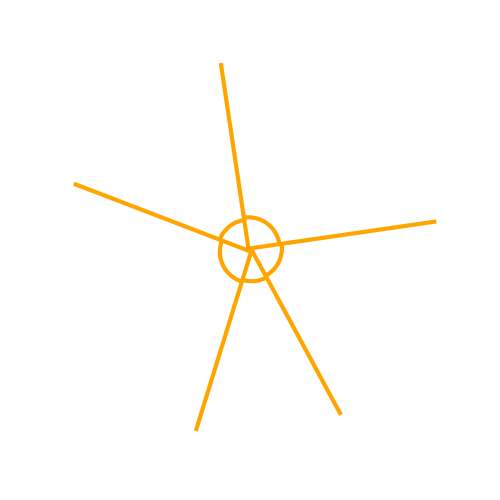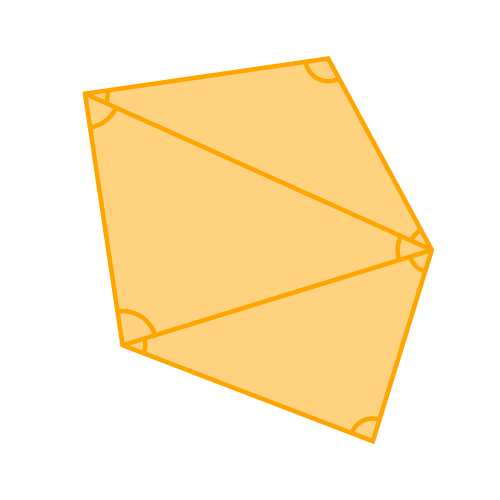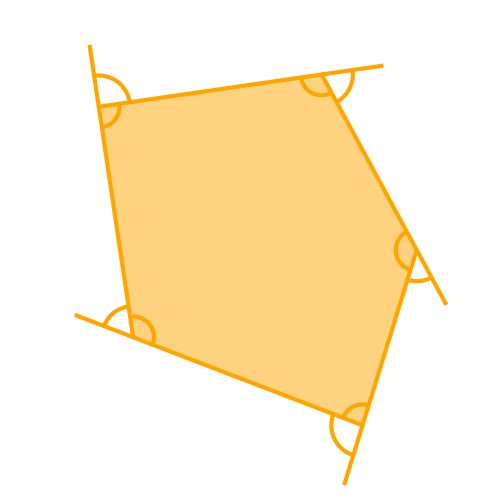# Interior and exterior angles of a polygon

Martin McBride
2020-08-16

Here are some basic geometry rules about the interior and exterior angles of a polygon. They are also covered in this video:

## Interior angles of a polygon

The angles inside a polygon are called its interior angles. Here is a 5-sided, irregular polygon, showing its interior angles:## Exterior angles of a polygon

If we extend one of the sides of a polygon, the angle between the extended side and the side next to it is called an exterior angle. If we do this for each side of the shape, we can al the exterior angles of the shape:## The sum of the exterior angles

The sum of the exterior angles in a polygon is always 360°. It doesn't matter how many side the shape has, the sum of the exterior angles is always the same.

To see why this is the case, imagine an ant crawling round the outside of the shape. Assume it goes in a clockwise direction. Each time it reaches a corner, the ant will turn to its right. It will turn through the exterior angle of that corner, so it faces in the correct direction to walk along the next side.

By the time the ant gets all the way around the shape it will have turned at each corner, so the total angle it has turned through will be the sum of the exterior angles of the shape. But after the ant has gone all the way around the shape it will be facing the same way as it was when it started, which means it has turned through 360°. Therefore the sum of the exterior angles in a polygon is 360°.

Here is another way to visualise this. Let's take the previous diagram and make the polygon smaller:Although the shape is smaller, it is still the same shape, so the exterior angles are still the same. Now lets make the shape very small, so you can hardly see it:Again, this illustrates that the sum of the exterior angles of a polygon is 360°.

## The sum of the interior angles

The sum of the interior angles is not quite so straightforward, but it is still quite a simple formula. It depends on the number of sides, n:

sum of interior angles = (n - 2) x 180


We can see how this is the case by dividing our shape into triangles, like this:In this case the original polygon has 5 sides, so we can divide it into 3 triangles. Every angle of every triangle contributes to one of the interior angles, and we know the rules of geometry that interior angles of a triangle add up to 180°. So the sum of the interior angles of a 5-sided shape is 3 x 180 (which fits in with the formula above).

So we know that:

• The interior angles of a triangle are 1 x 180 (from the rules of geometry).
• The interior angles of a quadrilateral are 2 x 180 (from the rules of geometry).
• The interior angles of a 5-sided polygon are 3 x 180 (shown above).

It is quite easy to show that a 6-sided shape can be split into 4 triangles, a 7-sided shape into 5 triangles, and so on, so the formula above applies to all polygons.

## Alternative proof

There is another way to look at interior and exterior angles that, of course, gives the same result in a different way.

Here is the same polygon with all the interior and exterior angles drawn:The important thing here is the interior and exterior angle at each corner form a straight line, in other words they add up to 180°.

This means that for an n-sided shape, the total of all the interior and exterior angles is:

n x 180°


And since we know that the total of the exterior angles in 360°, this means that the total of the interior angles is:

(n x 180°) - 360°


or:

(n - 2) x 180°


which is the formula given above.